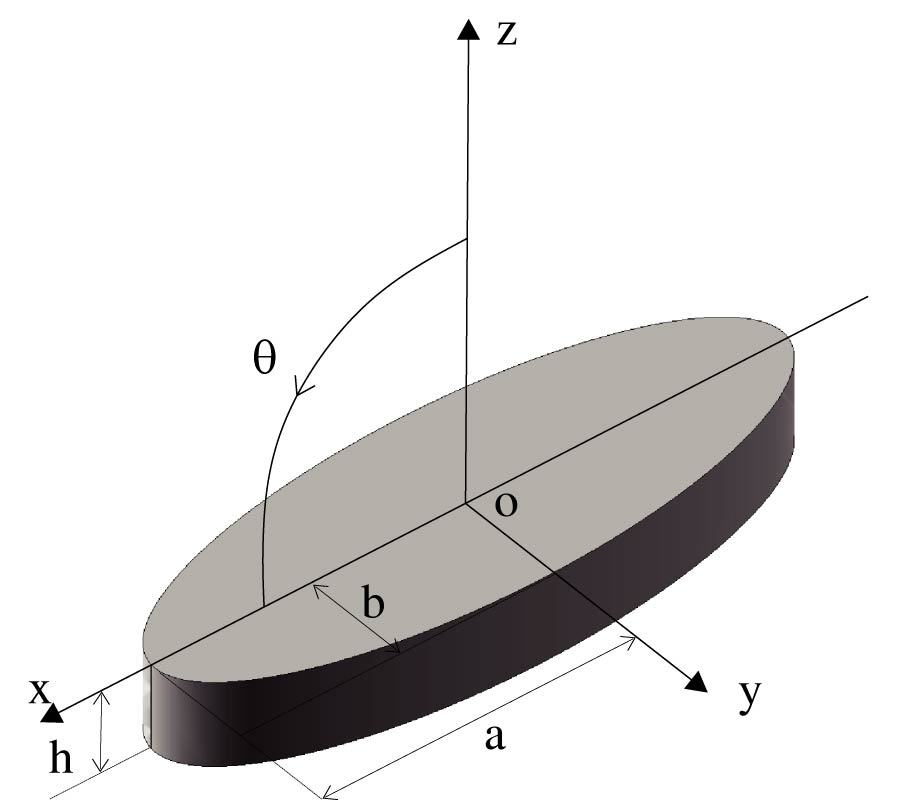# Vol. 43

Front:[PDF file] Back:[PDF file]
Latest Volume
All Volumes
All Issues
2015-08-11

#### Diffraction Calculation of Arbitrarily Curved Wedge Modeled with NURBS Surfaces

By Jun Yan, Jun Hu, Huapeng Zhao, and Zai-Ping Nie
Progress In Electromagnetics Research M, Vol. 43, 63-70, 2015
doi:10.2528/PIERM15070605

## Abstract

In this paper, we present an equivalent current-based numerical routine for calculating the diffraction of arbitrarily curved wedge modeled with non-uniform rational B-spline (NURBS) curves and surfaces. The NURBS curves and surfaces obtained from CAD systems need to be parameterized for numerical calculation; however, available parameterizing approaches in rendering computer graphics, which use straight line segments and flat facets for tessellation, are not suitable for the computation of the wedge diffraction. To make the full use of NURBS modeling technique in high-frequency asymptotic approaches, the proposed numerical routine utilizes a curvature adaptive tessellation scheme to parameterize the edge curve of the wedge with varying curvature as well as the method of parameter alignment to maintain the C0 continuity between the edge curve and the wedge surfaces, which is essential in evaluating the diffraction coefficients. Based on the proposed parameterizing method, the equivalent edge current can be implemented for diffraction computation of arbitrarily curved wedge modeled with NURBS curves and surfaces, complementing with the NURBS based physical optics (PO) as a fully NURBS-based high-frequency approach, which provides high geometrical accuracy and computational efficiency for calculating diffraction of electrically large curved wedges. Numerical examples are presented to validate the proposed method.

## Citation

Jun Yan, Jun Hu, Huapeng Zhao, and Zai-Ping Nie, "Diffraction Calculation of Arbitrarily Curved Wedge Modeled with NURBS Surfaces," Progress In Electromagnetics Research M, Vol. 43, 63-70, 2015.
doi:10.2528/PIERM15070605
http://www.jpier.org/PIERM/pier.php?paper=15070605

## References

1. Perez, J. and M. F. Catedra, "Application of physical optics to the RCS computation of bodies modeled with NURBS surface," IEEE Transactions on Antennas and Propagation, Vol. 42, No. 10, 1404-1411, 1994.
doi:10.1109/8.320747

2. Dominingo, M., F. Rivas, J. Perez, R., P. Torres, and M. F. Catedra, "Computation of the RCS of complex bodies modeled using NURBS surfaces," IEEE Antennas and Propagation Magazine, Vol. 37, No. 6, 36-47, 1995.
doi:10.1109/74.482030

3. Zhao, Y., X.-W. Shi, and L. Xu, "Modeling with NURBS surfaces used for the calculation of RCS," Progress In Electromagnetics Research, Vol. 78, 49-59, 2008.
doi:10.2528/PIER07082903

4. De Adana, F. S., I. G. Diego, O. G. Blanco, P. Lozano, and M. F. Catedra, "Method based on physical optics for the computation of the radar cross section including diffraction and double effects of metallic and absorbing bodies modeled with parametric surfaces," IEEE Transactions on Antennas and Propagation, Vol. 52, No. 12, 3295-3303, 2004.
doi:10.1109/TAP.2004.836444

5. Wang, N. X., J. Dang, H. B. Yuan, and C. H. Liang, "Study on curved wedge diffraction in NURBS-UTD method," Microwave and Optical Technology Letters, Vol. 55, No. 10, 2317-2321, 2013.
doi:10.1002/mop.27868

6. Balazs, A., M. Guthe, and R. Klein, "Efficient trimmed NURBS tessellation," Journal of WSCG, Vol. 12, No. 1–3, 2004.

7. Chen, X., S. Y. He, D. F. Yu, H. C. Yin, W. D. Hu, and G. Q. Zhu, "Geodesic computation on NURBS surfaces for UTD analysis," IEEE Antennas and Wireless Propagation Letters, Vol. 12, 194-197, 2013.
doi:10.1109/LAWP.2013.2245291

8. Michaeli, A., "Equivalent edge currents for arbitrary aspects of observation," IEEE Transactions on Antennas and Propagation, Vol. 32, No. 3, 252-258, 1984.
doi:10.1109/TAP.1984.1143303

9. Boehm, W., "Generating the Bezier points of B-spline curves and surfaces," Computer Aided Design, Vol. 13, No. 32, 356-366, 1981.

10. Farin, G., Curves and surface for CAGD, Morgan Kaufmann, San Francisco, 2004.

11. Yan, J., J. Hu, and Z. P. Nie, "Calculation of the physical optics scattering by trimmed NURBS surfaces," IEEE Antennas and Wireless Propagation Letters, Vol. 13, 1640-1643, 2014.
doi:10.1109/LAWP.2014.2348564